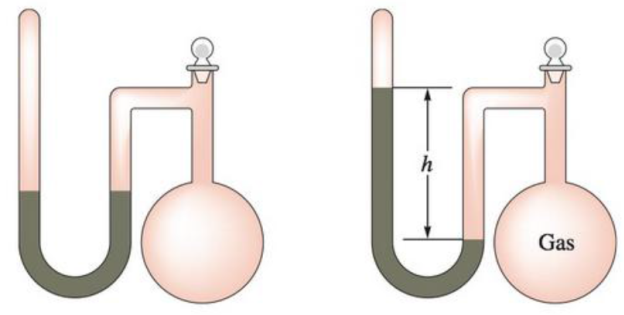# A sealed-tube manometer (as shown below) can be used to measure pressures below atmospheric pressure. The tube above the mercury is evacuated. When there is a vacuum in the flask, the mercury levels in both arms of the U-tube are equal. If a gaseous sample is introduced into the flask, the mercury levels are different. The difference h is a measure of the pressure of the gas inside the flask. If h is equal to 6.5 cm, calcúlate the pressure in the flask in torr, pascals, and atmospheres.### Chemistry: An Atoms First Approach

2nd Edition
Steven S. Zumdahl + 1 other
Publisher: Cengage Learning
ISBN: 9781305079243

#### Solutions

Chapter
Section### Chemistry: An Atoms First Approach

2nd Edition
Steven S. Zumdahl + 1 other
Publisher: Cengage Learning
ISBN: 9781305079243
Chapter 8, Problem 39E
Textbook Problem
4169 views

## A sealed-tube manometer (as shown below) can be used to measure pressures below atmospheric pressure. The tube above the mercury is evacuated. When there is a vacuum in the flask, the mercury levels in both arms of the U-tube are equal. If a gaseous sample is introduced into the flask, the mercury levels are different. The difference h is a measure of the pressure of the gas inside the flask. If h is equal to 6.5 cm, calcúlate the pressure in the flask in torr, pascals, and atmospheres.Interpretation Introduction

Interpretation:

The pressure of the given gas should be determined in units of torr, pascals and atm when the manometer shows a reading of 6.5cm.

Concept Introduction:

The manometer is a devise used measure the pressure of a gas. The pressure of gas is determined by the value of ‘h’ shown by the manometer. This ‘h’-value is added or subtracted with atmospheric pressure to determine the pressure of gas.

If the flask side mercury level is decreased after the filling of gas, then the ‘h’-value will be added to atmospheric pressure to get the pressure of gas.

Atmospheric pressure = 760 mm Hg /torr

The pressure equivalent of ‘h’ value is,

h=1cm=10mmHg

Pressure of a substance can be stated in various units. The units of pressure are interconvertible. The relations between units of pressure are,

• Since the unit mm Hg and the unit torr is used interchangeably.

1mmHg = 1torr

• The 1 mm Hg pressure in Pa unit is,

1mmHg = 101325760Pa

• Conversion of 1 torr into atm is,

1mmHg = 1760atm

### Explanation of Solution

Explanation

The given ‘h’ value for the gas in manometer is 6.5cm. The picture of manometer shows the    flask side mercury level is decreased after the filling of gas.

Hence the equation for finding the pressure of gas is,

Atmospheric pressure+h(inmm)

That is,

= (760+65)mmHg

= 825mmHg

The relations between units of pressure are,

1mmHg = 1torr , 1mmHg = 101325760Pa 1mmHg = 1760atm

The mm Hg and torr units are used interchangeably,

Therefore,

825mmHg = 825torr

The calculated pressure is 825mm Hg

### Still sussing out bartleby?

Check out a sample textbook solution.

See a sample solution

#### The Solution to Your Study Problems

Bartleby provides explanations to thousands of textbook problems written by our experts, many with advanced degrees!

Get Started

Find more solutions based on key concepts
The volume 0.250 L could also be expressed as mL.

Introductory Chemistry: A Foundation

Cite some properties that are characteristic of a metal.

General Chemistry - Standalone book (MindTap Course List)

Vitamin D functions as a hormone to help maintain bone integrity. T F

Nutrition: Concepts and Controversies - Standalone book (MindTap Course List)

What is a chemical reaction?

Biology: The Dynamic Science (MindTap Course List)

How can errors in the cell cycle lead to cancer in humans?

Human Heredity: Principles and Issues (MindTap Course List)

Match the term listed in Column A with its definition from Column B

Nutrition Through the Life Cycle (MindTap Course List)

A standing wave is established in a 120-cm-long string fixed at both ends. The string vibrates in four segments...

Physics for Scientists and Engineers, Technology Update (No access codes included)

What is the origin of oozes? What are the two types of oozes?

Oceanography: An Invitation To Marine Science, Loose-leaf Versin